# 2015年成人高考高起点数学(文史财经类)真题及答案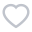472人已考
•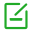卷面总分：150
•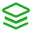试卷类型：历年真题
•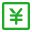测试费用： 免费
•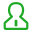关注人数：2237
•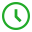作答时间：120分钟
•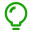解析：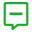试卷简介：
2015年成人高考高起点数学(文史财经类)真题及答案，本试卷为2015年成人高考(高起点)数学真题及答案，内包含答案及详细解析。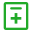试题类型：
• 一、选择题
• 二、填空题
• 三、解答题• 一、选择题
• 二、填空题
• 三、解答题
1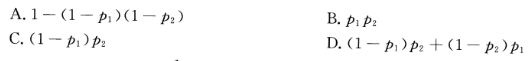A.A

B.B

C.C

D.D

2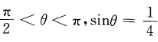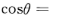A.A

B.B

C.C

D.D

3

A.4

B.-4

C.-1

D.1

4

A.{2,5,6}

B.{8}

C.{6}

D.{2,5,6,8}

5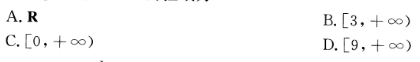A.A

B.B

C.C

D.D

6

A.A.-4

B.B.4

C.C.1

D.D.-1

7

A.27

B.1/9

C.1/3

D.3

8

A.y=1+2x

B.y=1—x

C.y=1+x2

D.y=1+Z-x

9

A.A.甲是乙的充分必要条件

B.B.甲是乙的必要条件，但不是乙的充分条件

C.C.甲是乙的充分条件，但不是乙的必要条件

D.D.甲不是乙的充分条件，也不是乙的必要条件

10

A.x-y+2=0

B.x+y-2=0

C.x+y+2=0

D.x-y=0